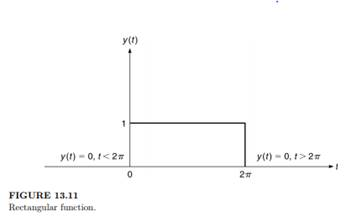Create an Account

Home / Questions / 1 Find the third Fourier coefficient of the function pictured in Figure 1310 in which

1 Find the third Fourier coefficient of the function pictured in Figure 1310 in which

1. Find the third Fourier coefficient of the function pictured in Figure 13.10, in which h = 1.

2. What is the value of the power spectrum at the cyclic frequency 1 Hz for the function given by the Figure 13.11? Give the answer to three significant figures. (The desired result is achieved by representing the function on an infinite interval.)Jul 26 2020 View more View LessSubscribe To Get Solution